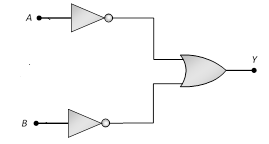## Physics MCQ on Digital Electronics for NEET, JEE, Medical and Engineering Exam 2021

MCQ on Digital Electronics: Previous trends reveal that NEET/JEE aspirants find Physics as the most challenging section amongst the other sections. But most of them fail to decipher the reason behind this. This particularly can be as the candidates taking up this examination are predominantly medical aspirants with their subject of interest being Biology. Also, sections like Physics are more on theories, laws, numerical as opposed to Biology which is more of fact-based, life sciences and comprising of substantial explanations.In this post we are providing you MCQ on Digital Electronics, which will be beneficial for you in upcoming NEET, AIIMS, JEE, WBJEE, Medical and Engineering Exams.

## MCQ on Digital Electronics

Q1.  The logic behind NOR gate is that it gives
a) High output when both the inputs are low
b) Low output when both the inputs are low
c) High output when both the inputs are high
d)  None of the above

(a) High output when both the inputs are low

Q2. A gate in which all the inputs must be low to get a high output is
a) NAND gate
b) Inverter
c) NOR gate
d) AND gate

(b) and (c) Inverter and NOR gate

Q3. Which logic gate is represented by the following combination of logic gates?a) OR
b) NAND
c) AND
d) NOR

(b) NAND

Q4. The Output of OR gate is 1
a) If both inputs are zero
b) If either or both inputs are 1
c) Only if both inputs are 1
d) If either input is zero

(b)  If either or both inputs are 1

Q5. Boolean Algebra is essentially based on
a) Truth
b) Logic
c) Symbol
d) Number

(b) Logic

Q6.  The outputs of a NAND gate is 0
a) If both inputs are 0
b) If one input is 0 and the other input is 1
c) If both inputs are 1
d) If both inputs are 1 or one of the inputs is 1 and the other one is 0

(c) If both inputs are 1

Q7. A logic gate is an electronic circuit which
a) Makes logic decisions
b) Allows electrons flow only in one direction
c) Works binary algebra
d) Alternates between 0 and 1 values

(a) Makes logic decisions

Q8. The following truth table corresponds to the logic gate

 Input Output
 A B X 0 0 0 0 1 1 1 1 1 1 0 1

a) NAND
b) OR
c) AND
d) XOR

(b) OR

Q9. Which of the following logic gate is a universal gate?
a) OR
b) NOT
c) AMND
d) NOR

(d) NOR

Q10. How many NAND gates are used to form an AND gate?
a) 1
b) 2
c) 3
d) 4

(b) 2